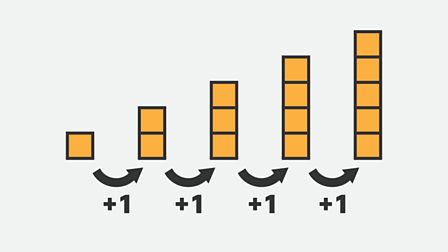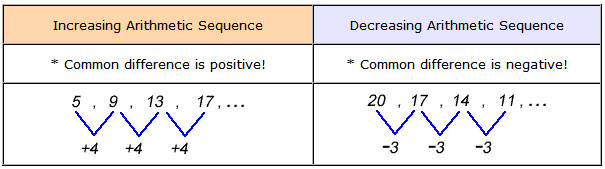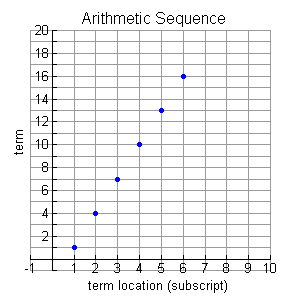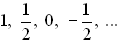Math Concepts

# How to find Common Difference?

20.7k views

 1 Introduction 2 How to find common difference 3 Common Difference Problems: Finding Common Ratio 4 Summary 5 FAQs 6 External References

18th September 2020

## Introduction

Did you know your birthday is just a sequence?

A sequence is defined as an arrangement of numbers in a particular order.

One example  sequence in real life is the celebration of people's birthday. The common difference between consecutive celebrations of the same person is one year.An arithmetic sequence goes from one term to the next by always adding (or subtracting) the same amount.

The number added (or subtracted) at each stage of an arithmetic sequence is called the "common difference" d, because if we subtract (that is, if you find the difference of) successive terms, you'll always get this common value.

## How to find common difference?

The common difference is the value between each number in an arithmetic sequence.

Therefore, you can say that the formula to find the common difference of an arithmetic sequence is: d = a(n) - a(n - 1), where a(n) is the last term in the sequence, and a(n - 1) is the previous term in the sequence.

There are two kinds of arithmetic sequence, one is increasing arithmetic sequence and the other is decreasing arithmetic sequence.Some sequences are made up of simply random values, while others have a fixed pattern that is used to arrive at the sequence's terms.

The arithmetic sequence (or progression), for example, is based upon the addition of a constant value to reach to  the next term in the sequence. The number being added to each term is constant (always the same).

a1,  (a1 + d),  (a1 + 2d),   ...

The fixed amount is called the common difference, d, referring to the fact that the difference between two successive terms generates the constant value that was added.

To find the common difference, subtract the first term from the second term.Arithmetic sequences have a linear nature when plotted on graph (as a scatter plot).

The domain consists of the counting numbers 1, 2, 3, 4,5 ... (representing the location of each term) and the range consists of the actual terms of the sequence.

In the graph shown above, while the x-axis increased by a constant value of one, the y value increased by a constant value of 3.

### Examples: How to find common difference?

Let's make an arithmetic sequence with a starting number of 2 and a common difference of 5.

Our first term will be our starting number:2

Our second term will be equal to the first term (2) plus the common difference (5); so our second term is 7.

So the first two terms of our sequence are:2, 7

Our third term will be equal to the second term (7) plus the common difference (5); so our third term is 12.

So the first three terms of our sequence are: 2, 7, 12

Our fourth term will be equal to the third term (12) plus the common difference (5); so our fourth term is 17.

So the first four terms of our sequence are: 2, 7, 12, 17

Now that you're familiar with making an arithmetic sequence from a starting number and a common difference, the problem you're doing is asking you to find the common difference of a given sequence.

The first term here  is 2; so that is your starting number.

The second term is 7. To find the difference between this and the first term, we take 7 minus 2; that gives us 5. So the difference between the first and second terms is 5.

The second term is 7 and the third term is 12. To find the difference, we take 12 minus 5; that gives us 5 again.

So the common difference between each term is 5. Below there are some examples you need to solve this type of problem.

Arithmetic Sequence:
Common Difference, d:
1, 6, 11, 16, 21, 26, ... d = 5.  5 is added to each term to arrive at the next term. so ... the difference a2 - a1 = 5.
10, 8, 6, 4, 2, 0, -2, -4, -6, d = -2.  -2 is added to each term to arrive at the next term.so ... the difference a2 - a1 = -2.d = -½. A -½ is added to each term to arrive at the next term.so ... the difference a2 - a1 = -½.

## Common Difference Problems: Finding Common Difference

Let's see a few more examples…...

 Example 1

What is the common difference in this sequence?

−3,0,3,6,9,12,...

Solution:

The common difference is the distance between each number in the sequence. Notice that each number is 3 away from the previous number.12−9=3   9−6=3   6−3=3   3−0=3   0−(−3)=3

 So the common difference is 3.
 Example 2

What is the common difference in the following sequence?

3,11,19,27,35

Solution:

To find it, simply subtract the first term from the second term, or the second from the third, or so on...
11−3=8
19−11=8
See how each time we are adding 8 to get to the next term? This means our common difference is

 8
 Example 3

What is the common difference in the following sequence?

\begin{align}\frac{{ - 3}}{4}\frac{{ - 1}}{4}\frac{1}{4}....\end{align}

Solution:

The common difference in this set is the linear amount spaced between each number in the set.
Subtract the first number from the second number.

\begin{align}\frac{1}{4} - \left( {\frac{{ - 3}}{4}} \right) = \frac{{ - 1}}{4} + \frac{3}{4} = \frac{2}{4} = \frac{1}{2}\end{align}

Check this number by subtracting the second number from the third number.

\begin{align}\frac{1}{4} - \left( {\frac{{ - 1}}{4}} \right) = \frac{{ - 1}}{4} + \frac{1}{4} = \frac{2}{8} = \frac{1}{2}\end{align}
Each spacing, or common difference is:

 \begin{align}\frac{1}{2}\end{align}

## Summary

We understand from the above examples that one of the two simplest sequences to work with is an arithmetic sequence .

It are used throughout mathematics .

It has important applications in physics, engineering, biology, economics, computer science, queueing theory, and finance

A key part of arithmetic sequence is its common difference. The above blog showcased using examples how to find it in various scenarios.

Cuemath, a student-friendly mathematics platform, conducts regular Online Live Classes for academics and skill-development and their Mental Math App, on both iOS and Android, is a one-stop solution for kids to develop multiple skills. Know more about the Cuemath fee here, Cuemath Fee

## What is common difference?

An arithmetic sequence is a sequence with the difference between two consecutive terms constant. The difference is called the common difference.

## How to find the common difference?

The common difference is the value between each number in an arithmetic sequence. ... Therefore, you can say that the formula to find the common difference of an arithmetic sequence is: d = a(n) - a(n - 1), where a(n) is the last term in the sequence, and a(n - 1) is the previous term in the sequence.## Increasing arithmetic difference?

Common difference is positive

## Decreasing arithmetic difference?

Common difference is negative.

## External References

Related Articles
GIVE YOUR CHILD THE CUEMATH EDGE
Access Personalised Math learning through interactive worksheets, gamified concepts and grade-wise courses